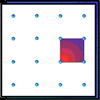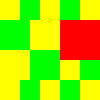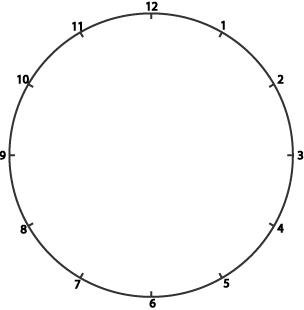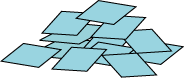#### You may also like### Geoboards

This practical challenge invites you to investigate the different squares you can make on a square geoboard or pegboard.### Tiles on a Patio

How many ways can you find of tiling the square patio, using square tiles of different sizes?### Pebbles

Place four pebbles on the sand in the form of a square. Keep adding as few pebbles as necessary to double the area. How many extra pebbles are added each time?

# A Square in a Circle

##### Age 7 to 11Challenge Level

Harry had a circle which was marked with twelve numbered dots to help him draw clock faces. The circle had a diameter of $10$ cm.Harry drew lines from the $12$ to the $3$, from the $3$ to the $6$, from the $6$ to the $9$, and then back from the $9$ to the $12$.